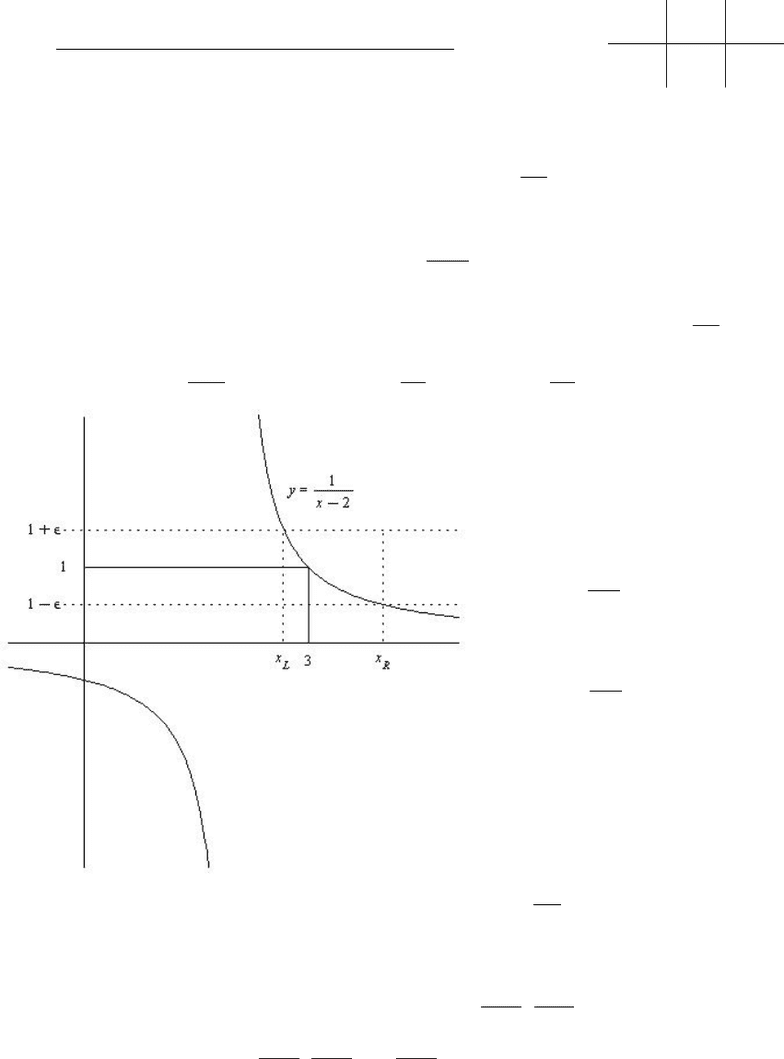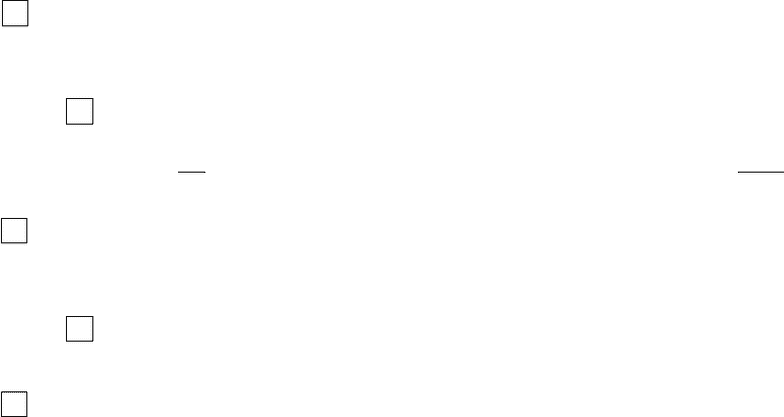# MTH 151 Study Guide - Quiz Guide: 2 On

52 views5 pagesName:
Exam 1 - Math 151
Sep 11, 2007
R. Ketchersid
1. [9pts] a) [3pts] Give the rigorous (“ε-δ”) deﬁnition of “limx31
x2= 1”.
For all ε > 0, there is a δ > 0such that
0<|x3|< δ =
1
x21
< ε
b) [3pts] Find an interval (xL, xR)(for arbitrary ε) satisfying xL< x < xR1ε < 1
x2<1 + ε.
(See graph.)
For xLyou must solve 1
xL2= 1 + εso xL2 = 1
1+εand xL= 2 + 1
1+ε. Similarly compute xR.
xL= 2 + 1
1+ε
xR= 2 + 1
1ε
c) [3pts] Use the above to give a δεsatisfying 0<|x3|< δε
1
x21< ε.
δε= min{3xL, xR3}= min 11
1 + ε,1
1ε1
= min ε
1 + ε,ε
1ε=ε
1 + ε
Use your δεto ﬁnd: (Give actual numbers with at least 4 decimal places of accuracy.)
δ1= 0.5δ0.1= 0.0909 δ0.01 = 0.0099
Unlock document

This preview shows pages 1-2 of the document.
Unlock all 5 pages and 3 million more documents.-2-
On problems 2 and 3, Tmeans the fact is necessarily true, Fmeans the fact is necessarily false,
and Nmeans that there is not enough information, that is the fact could be true or false in various
cases.
2. [5pts] Suppose f(x)is continuous on [0,)and f(0) = 1 and limx→∞ = 0.
a) T F N f(x)has a maximum on [0,).
There is some x0so that for xx0,|f(x)|<1/2since limx→∞ f(x)=0. By extreme value theorem
f(x)has a maximum value Mon [0, x0].M1so Mis the maximum value of f(x)on [0,).
b) T F N f(x)has a minimum on [0,).
It is easy to imagine graphs, to come up with expressions requires a bit more thought. The
following work: f(x) = 1
x+1 clearly shows that there need not be a minimum while f(x) = 1x
(x+1)2
has a minimum.
c) T F N There is some c > 0such that f(c)=1/2.
This is IVT. Since limx→∞ f(x)=0we have some x > 0so that f(x)<1/2. By IVT there is
c(0, x)with f(c) = 1/2.
d) T F N There is some c > 0such that f(c) = 0.
Same examples as in (b) work.
e) T F N There is some c > 0such that limxcf(x)=1/3.
Just as in (c) there is some cso that f(c) = 1/3. since fis continuous at c,limxcf(x)=1/3.
3. Problem 3 was thrown out for those students taking the version of the exam with T/F/N. The
problem should have read “g(x)is continuous at x= 4 as written it does not really make sense.
See next page for intended solution.
Unlock document

This preview shows pages 1-2 of the document.
Unlock all 5 pages and 3 million more documents.

## Document Summary

Sep 11, 2007: ketchersid, [9pts] a) [3pts] give the rigorous ( - ) de nition of limx 3. For all > 0, there is a > 0 such that. 1 (cid:12)(cid:12)(cid:12)(cid:12) (cid:12)(cid:12)(cid:12)(cid:12) < : [3pts] find an interval (xl, xr) (for arbitrary ) satisfying xl < x < xr 1 < 1 (see graph. ) For xl you must solve xl 2 = 1 + so xl 2 = 1. Similarly compute xr. x 2 < 1 + . 1 : [3pts] use the above to give a satisfying 0 < |x 3| < (cid:12)(cid:12)(cid:12) 1 x 2 1 (cid:26) (cid:27) = min{3 xl, xr 3} = min. 1 + (cid:12)(cid:12)(cid:12) < . (cid:27) (cid:26) Use your to nd: (give actual numbers with at least 4 decimal places of accuracy. ) There is some x0 so that for x x0, |f(x)| < 1/2 since limx f(x) = 0. By extreme value theorem f(x) has a maximum value m on [0, x0].